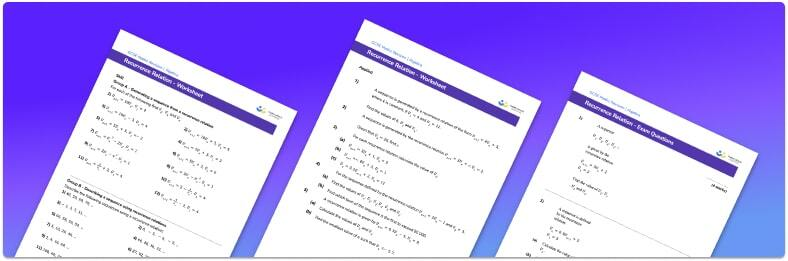# Recurrence relation worksheet• Section 1 of the recurrence relation worksheet contains 36 skills-based recurrence relation questions, in 3 groups to support differentiation
• Section 2 contains 5 applied recurrence relation questions with a mix of worded problems and deeper problem solving questions
• Section 3 contains 3 foundation and higher level GCSE exam style recurrence relation questions
• Answers and a mark scheme for all recurrence relation questions are provided
• Questions follow variation theory with plenty of opportunities for students to work independently at their own level
• All questions created by fully qualified expert secondary maths teachers
• Suitable for GCSE maths revision for AQA, OCR and Edexcel exam boards

• This field is for validation purposes and should be left unchanged.

You can unsubscribe at any time (each email we send will contain an easy way to unsubscribe). To find out more about how we use your data, see our privacy policy.

### Recurrence relation at a glance

A recurrence relation is a relation which creates a sequence of numbers. Consecutive terms of a sequence are found using a rule which gives the next term based on the current term. An example of a linear recurrence relation is u_{n+1}=2u_{n}+3. If the first term is 5, the next terms of the sequence  are found by multiplying each previous term by 2 and then adding 3.

u_{0}=5

u_{1}=2×5+3=13

u_{2}=2×13+3=29 etc. . .

We might be asked to find an expression for u_{n}. This is the nth term formula for the sequence. A recurrence relation of the form u_{n+1}=u_{n}+a where the coefficient of u_{n} is 1 and a is a constant, will produce a linear sequence. An example of this is the following recurrence relation: u_{n+1}=u_{n}+5 with initial value u_{0}=1, which gives the following sequence: 1, 6, 11, 16, 21, …. In this case, we can find u_{n}=5n-4.

Looking forward, students can then progress to additional sequences worksheets or more  algebra worksheets, for example a sequences worksheet, or simultaneous equations worksheet.For more teaching and learning support on Algebra our GCSE maths lessons provide step by step support for all GCSE maths concepts.

## Do you have KS4 students who need more focused attention to succeed at GCSE?There will be students in your class who require individual attention to help them succeed in their maths GCSEs. In a class of 30, it’s not always easy to provide.

Help your students feel confident with exam-style questions and the strategies they’ll need to answer them correctly with our dedicated GCSE maths revision programme.

Lessons are selected to provide support where each student needs it most, and specially-trained GCSE maths tutors adapt the pitch and pace of each lesson. This ensures a personalised revision programme that raises grades and boosts confidence.

Find out more